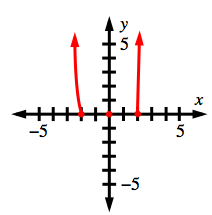### Home > INT3 > Chapter 10 > Lesson 10.2.2 > Problem10-126

10-126.

Sketch a graph of $y = x^3(x - 2)(x + 2)^2$

What are the $x$- and $y$-intercepts?
How does the function behave at each $x$-intercept?

What is the end behavior of the function?